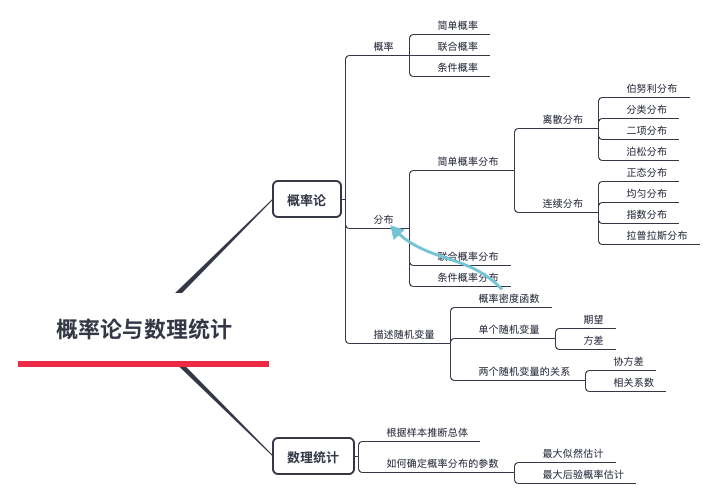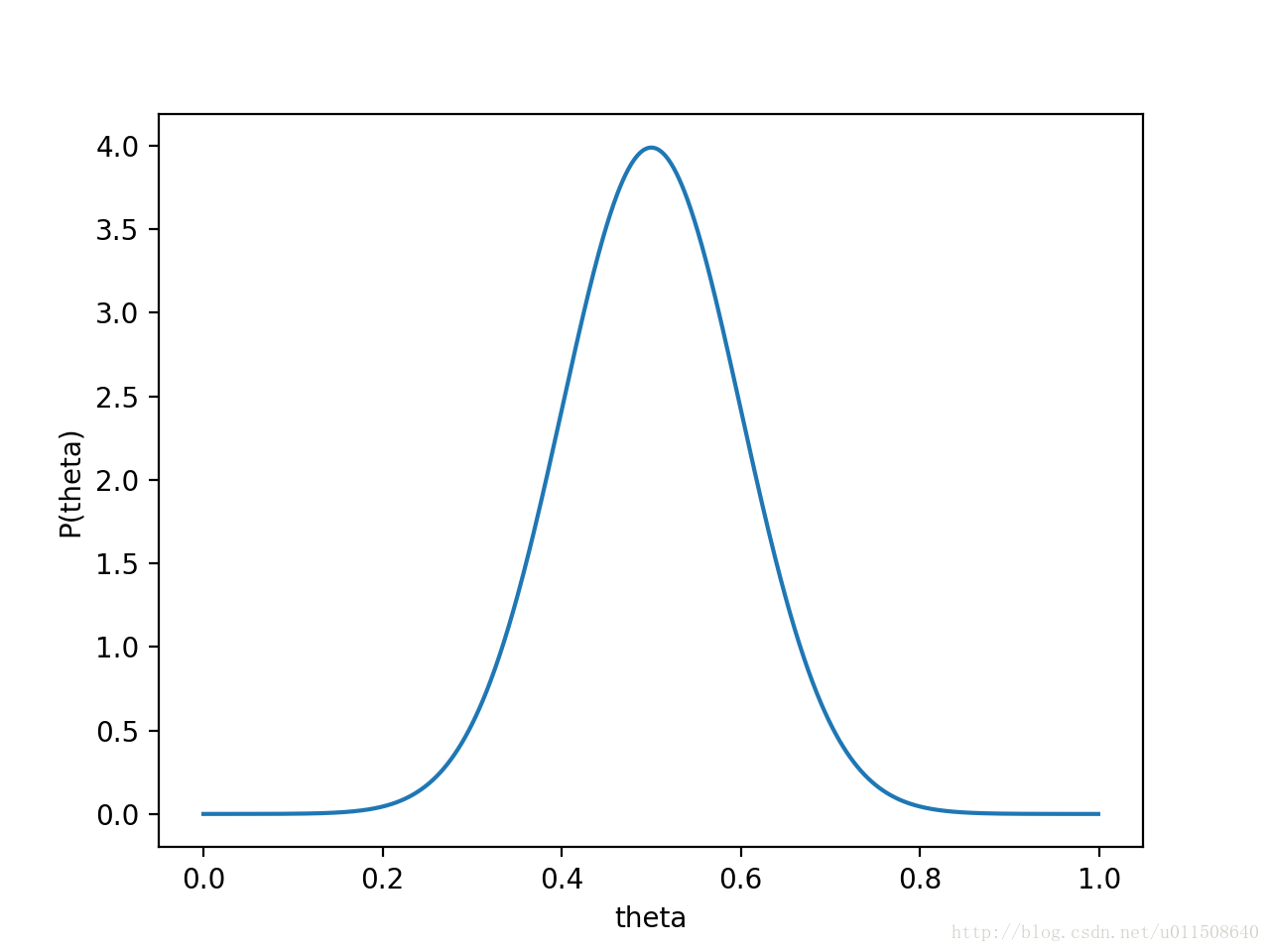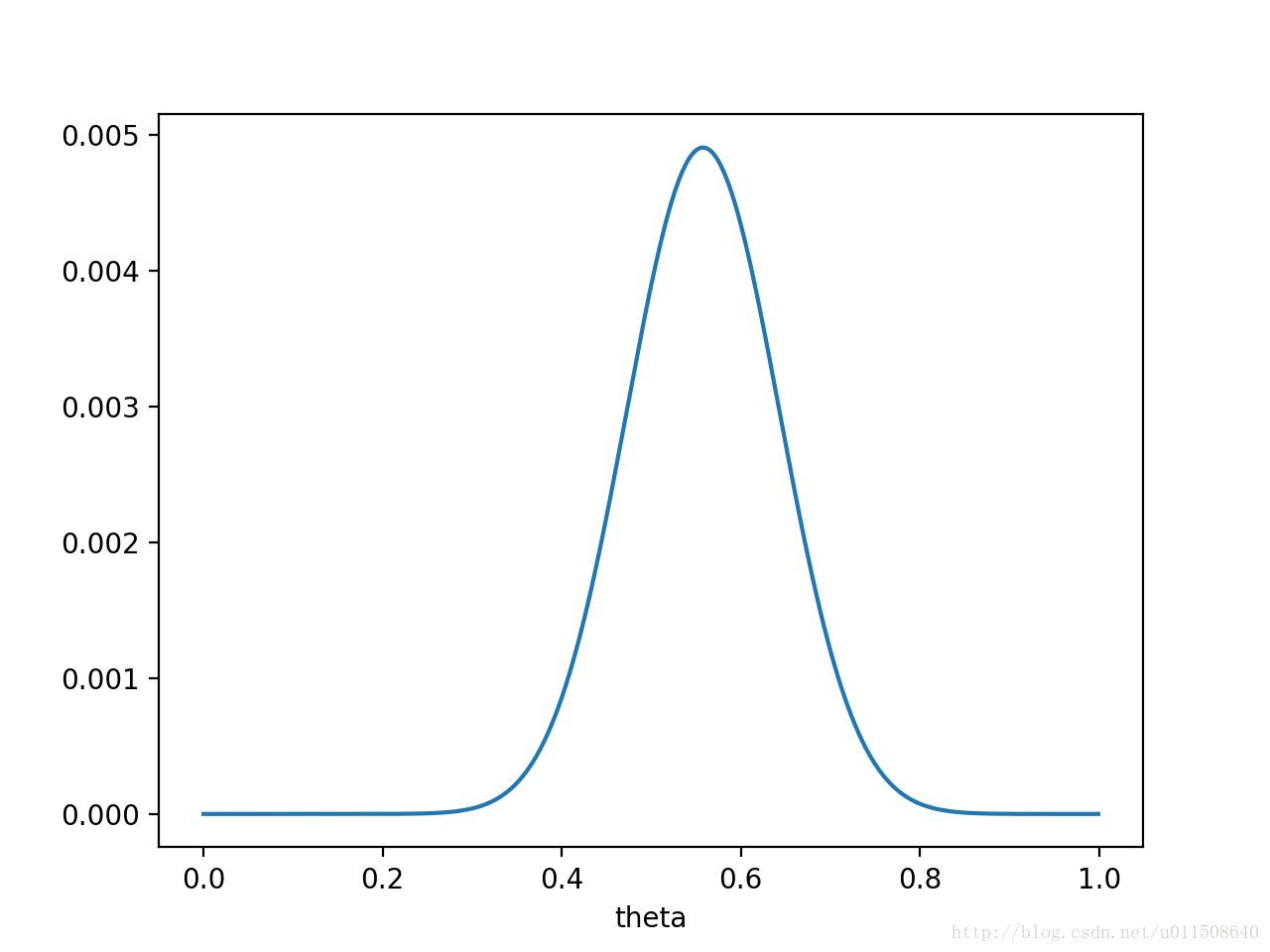2019年08月31日

## 简介

《成为AI产品经理》在概率统计中，我们最需要掌握的就是概率的分布。举个例子，我们在做一个预测用户评分的时候，这个分数可能是购买倾向，也可能是信用评分。按照经验，这个评分结果应该是符合正态分布的。这个时候，如果算法同学的模型预测出来的结果不符合正态分布，我们就必须对这个结果进行质疑，让他们给出合理的解释。从这个例子中我们知道，概率分布是我们用来评估特征数据和模型结果的武器。那产品经理怎么才能利用好这个武器呢？首先，我们要掌握常用的概率分布的类型。其次，我们还要知道业务场景下的特征数据和模型结果的分布，以及它们应该符合哪种分布类型。这样，产品经理就可以把概率分布应用于日常的工作中。

## 概率论### 贝叶斯公式的另类解读

P(AB) = P(A|B) * P(B) = P(B|A) * P(A)

$P(A|B) = \frac{P(B|A) * P(A)}{P(B)} = \frac{P(B|A)}{P(B)} * P(A)$

P(A|B) 可以视为 P(A) 的增强，也就是后验概率是先验概率的增强。观测者观测历史数据得出预测假设P(A)，然后新的信息P(B)出现，观测者修正预测为P(A|B)P(B|A)/P(B) 被称为调整因子。

### 概率论与数理统计

概率论 数理统计

1. 如果θ是已知确定的，x是变量，这个函数叫做概率函数(probability function)，它描述对于不同的样本点x，其出现概率是多少。
2. 如果x是已知确定的，θ是变量，这个函数叫做似然函数(likelihood function), 它描述对于不同的模型参数，出现x这个样本点的概率是多少，也可以用P(x;θ) 来表示

1. 最大似然估计是求参数θ, 使似然函数P(data|θ)最大。换个说法，求使似然函数P(data|θ)最大的θ
2. 最大后验概率估计则是想求θ使P(data|θ)P(θ)最大。换个说法，求使P(data|θ)P(θ)最大的θ

### 最大似然估计MLE

$P(data|θ)=(1−θ)×θ×θ×θ×θ×(1−θ)×θ×θ×θ×(1−θ)=θ^7(1−θ)^3=f(θ)$

### 最大后验概率估计MAP

MAP其实是在最大化

$P(θ|data)=\frac{P(data|θ)P(θ)}{P(data)}$$$P(data θ)P(θ)=θ^7(1−θ)^3P(θ)$$，则P(data|θ)P(θ)的函数图像为：1. 如果先验认为这个硬币是概率是均匀分布的，被称为无信息先验( non-informative prior )，通俗的说就是“让数据自己说话”，此时贝叶斯方法等同于频率方法。
2. 随着数据的增加，先验的作用越来越弱，数据的作用越来越强，参数的分布会向着最大似然估计靠拢。而且可以证明，最大后验估计的结果是先验和最大似然估计的凸组合。